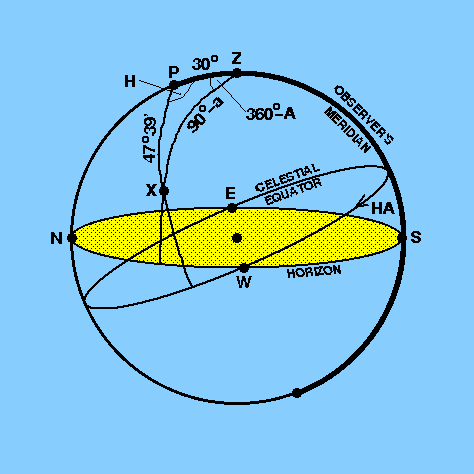Here, we will attempt a very common problem in spherical astronomy, which is also probably the most important one of all to understand - the conversion of equatorial coordinates into horizontal coordinates.

 1 A star, X, of declination= 42°21´N is observed when its hour angle, H = 8h16m42s. If the observer's latitude,, is 60°N, calculate the star's altitude, a and azimuth, A, at the time of observation.
The most important step is to sketch as accurately as possible a celestial sphere of the problem, as shown in Figure 25.

 figure 25: Example problem 1 - the conversion of equatorial coordinates to horizontal coordinates.Figure 25 shows that the spherical triangle we need to work with is triangle PZX. We have:

PX = 90° -= 90° - 42°21´ = 47°39´,
ZPX = H = 8h16m42s = 124°10´30´´,   and
PZ = 90° -= 90° - 60° = 30°.

Applying the cosine formula, we obtain

cos ZX = cos PZ cos PX + sin PZ sin PX cos ZPX

and hence

cos ZX = cos 30° cos 47°39´ + sin 30° sin 47°39´ cos 124°10´30´´.

Recalling that, for any angle x,

sin x = cos (90° - x)   and
cos x = sin (90° - x),

we can write

sin a = cos 30° cos 47°39´ + sin 30° sin 47°39´ cos 124°10´30´´,

which gives

a = 22°04´34´´.

To determine the azimuth we can apply the sine formula to triangle PZX, which gives

sin H / sin (90° - a) = sin (360° - A) / sin 47°39´,   or

sin (360° - A) = sin H sin 47°39´ / cos a.

Thus

sin (360° - A) = sin 124°10´30´´ sin 47°39´ / cos 22°04´34´´.

The sine formula must be used with care since it is not possible to say whether A is acute or obtuse, unless other information is available, i.e. the sine formula gives A or 180° - A. Hence we have

360° - A = 41°17´6´´ or 138°42´54´´,

that is

A = 318°42´54´´ or 221°17´6´´.

An inspection of Figure 25 suggests that the former answer is correct, but we should check it anyway. We can do this by using the cosine formula again:

cos 47°39´ = cos 30° cos (90° - a) + sin 30° sin (90° - a) cos (360° - A)

cos 47°39´ = cos 30° sin 22°04´34´´ + sin 30° cos 22°04´34´´ cos (360° - A).

This gives

360° - A = 41°17´6´´   and so

A = 318°42´54´´ east of north.

Two things should be noted here. The first is that although the cosine of an angle, A, suffers from an ambiguity between A and 360°-A, it does not suffer from the acute/obtuse problem that the sine of an angle suffers from, which is why the above check works. Secondly, you may have wondered why the altitude we calculated above does not suffer from the acute/obtuse problem. Strictly speaking, it does, but because altitude is simply the angular distance of the star above the horizon, 180° - a is equivalent to a.